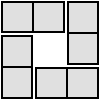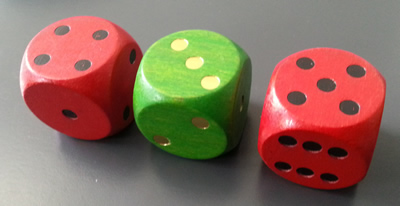#### You may also like### Consecutive Numbers

An investigation involving adding and subtracting sets of consecutive numbers. Lots to find out, lots to explore.### Domino Square

Use the 'double-3 down' dominoes to make a square so that each side has eight dots.### 4 Dom

Use these four dominoes to make a square that has the same number of dots on each side.

# Roll These Dice

## Roll These DiceTwo dice are RED and one is GREEN.

Add up the numbers on the two RED dice and then subtract the number on the GREEN.

So if one RED is 4 and the other RED is 5 and the GREEN is 3 we should add together 4 and 5 to make 9 and then subtract the 3 so that gives us a final answer of 6.

You'll need to roll these dice many times and see what numbers you make each time by doing the addition and subtraction.

In this game it would be good to find out:-

• what are the final answers by doing the addition and subtraction each time?
• what are all the different possible numbers?
• is there a good way of making sure you find them all?
• how will you record what you've found out?

Now have a go!

Look at your results and write down some questions that you could ask about them. For example, do any of them have the same answers? If so , why?

Then you could ask yourself, "I wonder what would happen if, instead, I ...?''

### Why do this problem?

This activity offers practice in addition and subtraction, including negative results, but the main aim is for pupils to concentrate on making sure that all the ways of rolling the three dice are reached. This will need some sort of system and you could focus on how answers could be recorded.

### Possible approach

You could introduce the problem using real dice and modelling the calculation a few times so that pupils get a feel for it. Once a few results have been recorded on the board, invite the pupils to speculate on how many different results there might be.

Ask them to work in pairs or small groups on the problem, saying very little else at this stage, but after a short time, bring them together again to share insights so far. Discuss the range of answers that learners have found up to that point and make sure they are happy with subtracting one number from a smaller number. (Using a number line which includes negative numbers might be helpful at this point.) Invite some pairs to describe how they are working. Some may be throwing real dice, others may be listing numbers. Encourage some sort of system so that they can be sure no results are left out. You could ask children to suggest ways of recording which would help - this could be in the form of a table or chart, but allow pupils to choose a way that suits them.

### Key questions

What are all the different possible numbers?
What are the final answers by doing the addition and subtraction each time?
Is there a good way of making sure you find all the possibilities?
How will you record what you've found out?

### Possible extension

Those children who are beginning to use the probability scale from $0$ to $1$, could begin to quantify the likelihood of getting particular results. Asking and investigating 'What if ...?' questions would be another good follow-up activity.

### For more extension work

Pupils can be challenged to use multiplication as well as addition and subtraction.  After some experimentation, they could try to predict totals that will NOT be possible, then check these predictions out, as well as explaining the predictions.

### Possible support

Most children will need dice to begin this activity.  For some the dice themselves are an essential prop. Some pupils will benefit from an adult working alongside them and asking questions along each step of the way until their confidence has increased.